1800-1023-196

+91-120-4616500

# Equivalent weight of Oxidising and Reducing Agents

## Equivalent weight of Oxidising and Reducing Agents

Equivalent weight of a substance (oxidant or reductant) is equal to molecular weight divided by number of electrons lost or gained by one molecule of the substance in a redox reaction.

Eq. wt. of O. A. =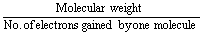=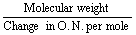Eq. wt. of R. A. =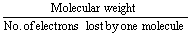=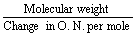Table : Equivalent weight of few oxidising/reducing agents

 Agents O. N. Product O. N. Change inO. N. peratom TotalChange inO. N. per mole Eq. wt.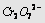+ 6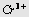+ 3 3 3 × 2 = 6 Mol. wt./6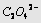+ 3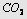+ 4 1 1 × 2 = 2 Mol. wt./2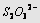+ 2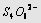+ 2.5 0.5 0.5 × 2 = 1 Mol. wt./1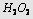– 1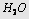– 2 1 1 × 2 = 2 Mol. wt./2– 1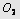0 1 1 × 2 = 2 Mol. wt./2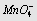(Acidic medium) + 7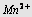+ 2 5 5 × 1 = 5 Mol. wt./5(Neutral medium) + 7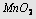+ 4 3 3 × 1 = 3 Mol. wt./3(Alkaline medium) + 7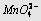+ 6 1 1 × 1 = 1 Mol. wt./1

## NEET & AIIMS Exam Sample Papers

 AIIMS SAMPLE PAPERS View More NEET SAMPLE PAPERS View More